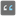• # Incomprehensible behaviour

## Question related to missionWords Order

Hi

I've got a function, which returns True or False: (mission Words Order)

```words_order=lambda t,w:w==[*(s:=set(w))]and s<=set(t:=t.split())and(a:=[*map(t.index,w)])==sorted(a)
```

The problem is that it gives different results for the same input. I assume, the problem hides in here

```w==[*(s:=set(w))]
```

but I don't understand why it is equality sometimes and the other times not

Any help is greatly appreciated!